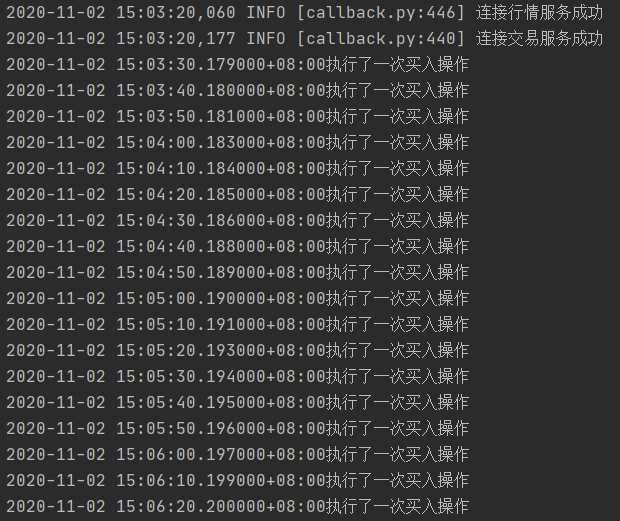【掘金使用技巧3】定时任务的多种可能Pinned highlighted四两 发表在策略研究 2020-11-02 15:46:48

834
0
0

# 定时任务的多种可能

## 每个月的第n天运行

def init(context):
# 获取全部交易日
end_date=context.backtest_end_time))
# 用来记录倍数的变量(索引为0时第一次运行，索引为1时第二次运行。。。)
context.k = 0
# 用来记录每隔几天运行一次
context.n = 3
# 定时任务
schedule(schedule_func=algo,date_rule='1d',time_rule='09:00:00')

# 每个月最后一天运行一次
def algo(context):
# 策略在每个月最后一天启动
now = context.now.month             # 保存当前月份
# 如果当前交易日对应的月份和下一个交易日对应的月份不同，则说明当前交易日是该月的最后一天
order_volume(symbol='SHSE.600519', volume = 100, side = 1, order_type = 2,position_effect=1)
print('{}执行了一次买入操作'.format(context.now))## 每n天运行一次（回测模式下）

def init(context):
# 获取全部交易日
end_date=context.backtest_end_time))
# 用来记录倍数的变量(索引为0时第一次运行，索引为1时第二次运行。。。)
context.k = 0
# 用来记录每隔几天运行一次
context.n = 3
# 定时任务
schedule(schedule_func=algo,date_rule='1d',time_rule='09:00:00')

# 每3天运行一次
def algo(context):
# 获取当前时间所在索引
now = str(context.now.date())

# 如果是第一个交易日，直接开仓
if now_index == 0:
order_volume(symbol='SHSE.600519', volume=100, side=1, order_type=2, position_effect=1)
print('{}执行了一次买入操作'.format(context.now))
context.k += 1
return

# 不是第一个交易日时，需要和策略执行信号放在一起比较
signal = context.k * context.n
if now_index == signal:
order_volume(symbol='SHSE.600519', volume=100, side=1, order_type=2, position_effect=1)
print('{}执行了一次买入操作'.format(context.now))
context.k += 1## 每n天运行一次（实时模式）

实时模式

def init(context):
while True:
time.sleep(10)
algo(context)

def algo(context):
order_volume(symbol='SHSE.600519', volume = 100, side = 1, order_type = 2,position_effect=1)
print('{}执行了一次买入操作'.format(context.now))

if __name__ == '__main__':
run(strategy_id='请输入你的策略id',
filename='main.py',
mode=MODE_LIVE,
token='请输入你的token')Looks like your connection to 掘金量化社区 - 量化交易者的交流社区 was lost, please wait while we try to reconnect.

• 提问
遇到问题？到社区提问，我们为您解答.
• 商业
咨询
有商业合作意向？请发邮件联系我们.
• QQ
加入掘金量化QQ群
• 公众号
关注掘金量化微信公众号
• TOP
返回顶部